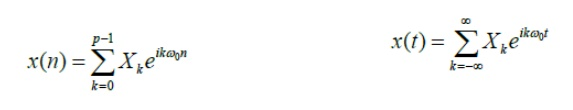Home | | Signals and Systems | Inverse Transforms

# Inverse Transforms

If we have the full sequence of Fourier coefficients for a periodic signal, we can reconstruct it by multiplying the complex sinusoids of frequency Žē0k by the weights Xk and summing:

Inverse Transforms

If we have the full sequence of Fourier coefficients for a periodic signal, we can reconstruct it by multiplying the complex sinusoids of frequency Žē0k by the weights Xk and summing:We can perform a similar reconstruction for aperiodic signalsThese are called the inverse transforms.

Study Material, Lecturing Notes, Assignment, Reference, Wiki description explanation, brief detail
Signals and Systems : Analysis of Continuous Time Signals : Inverse Transforms |